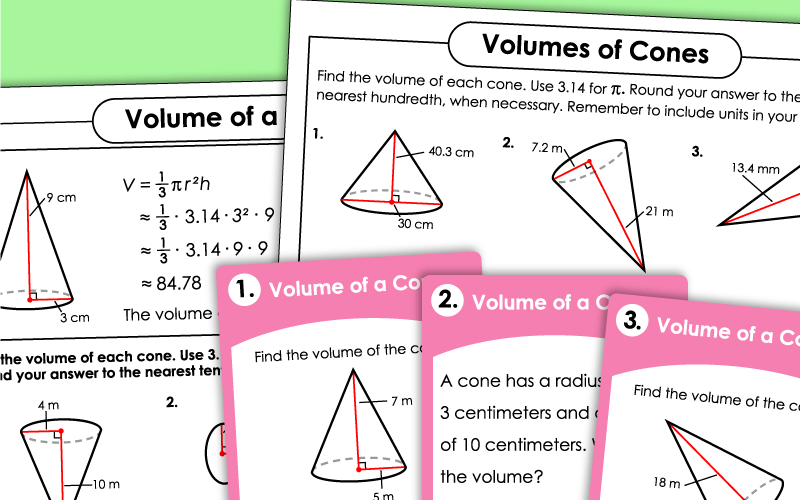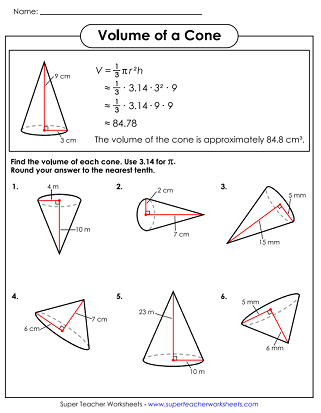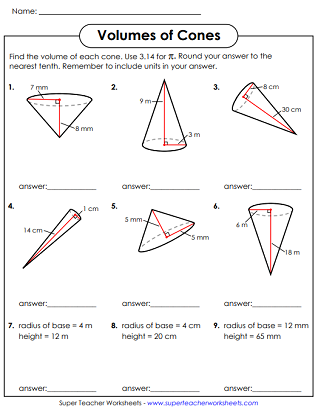# Volume of a Cone

This page has a collection printable worksheets for teaching students to calculate the volume of a cone.## Level: Basic

On this worksheet, students will calculate the volumes of six different cones. There's an example at the top to show them how it's done. Basic level means that measurements are not given as decimals.
On this page, students will calculate the volumes of nine different cones. There is a bit of room for students to show their work.
Print these 30 task cards on card stock. Cut them apart to use for peer study groups, small group instruction, morning work, or as a visual on your document camera.

At the top of this page is a model problem that shows students how to calculate the volume of a cone. Below are six problems for students to solve. Because this is an advanced-level worksheet, most problems have decimals in the given numbers.
Determine the volumes of nine different cones. One or more given measurements is a decimal.
More Volume Worksheets

Use this index to navigate to worksheets on volumes of rectangular prisms, cubes, cylinders, spheres, and prisms.

## Worksheet ImagesMy Account
Site Information